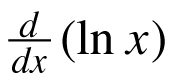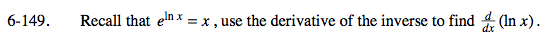### Home > CALC > Chapter 6 > Lesson 6.4.2 > Problem6-149

6-149.

Recall that elnx = x, use the derivative of the inverse to find. Homework Help ✎elnx = x is another way of saying that y = ex and y = lnx are inverse functions.
Inverse functions have reciprocal derivatives at their corresponding (x, y) → (y, x) values.

If f(x) has coordinate point (a, b).

$\text{And }f'(a)=\frac{2}{5}$

And if g(x) is the inverse of f(x),

$\text{then }g'(b)=\frac{5}{2}$

Let f(x) = ex and g(x) = lnx.
First find f '(x) = _____. (This should be easy!)

To find g'(x), take the reciprocal of f '(x), but NOT at the
x-value...

$g'(x)=\frac{1}{f'(y)}=\frac{1}{f'(g(x))}$

Now evaluate g'(x) when f(x) = ex.